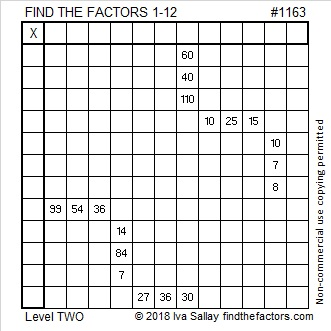# 1163 and Level 2

Can you write the numbers 1 to 12 in both the first column and the top row of this puzzle so those numbers and the clues function like a multiplication table? Sure you can!Print the puzzles or type the solution in this excel file: 12 factors 1161-1173

Here is some information about the number 1163:

• 1163 is a prime number.
• Prime factorization: 1163 is prime.
• The exponent of prime number 1163 is 1. Adding 1 to that exponent we get (1 + 1) = 2. Therefore 1163 has exactly 2 factors.
• Factors of 1163: 1, 1163
• Factor pairs: 1163 = 1 × 1163
• 1163 has no square factors that allow its square root to be simplified. √1163 ≈ 34.10279

How do we know that 1163 is a prime number? If 1163 were not a prime number, then it would be divisible by at least one prime number less than or equal to √1163 ≈ 34.1. Since 1163 cannot be divided evenly by 2, 3, 5, 7, 11, 13, 17, 19, 23, 29 or 31, we know that 1163 is a prime number.Prime number 1163 is also the sum of nine consecutive primes:
107 + 109 + 113 + 127 + 131 +137 + 139 + 149 + 151 = 1163

This site uses Akismet to reduce spam. Learn how your comment data is processed.